# What are Parametric Equations?

Parametric equations are math statements that describe a relationship between 2 items via a common third item.

## Examples of Parametric Equations

Parametric equations come in pairs.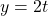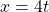## Understanding Parametric Equations

To understand parametric equations, you need to understand regular mathematical equations.

If you don’t understand regular equations, detour over here to learn them: What is an Equation?

Simplifying a parametric equation

If an apple costs 2 dollars and a banana costs 1 dollar. One apple can buy you two bananas.

Thus, in math terms, Apple = 2 Bananas.

I.e. Apple = 2 x Banana. Or in other words,

Apple = 2 Banana

Similarly, if an orange costs 4 dollars and a banana still costs 1 dollar.

Orange = 4 Banana

This tells us that we can get 2 apples for 1 orange.

2 Apple = Orange

Converting Fruits to Math

Let’s use a single letter to represent the names apple, banana and orange. I will call them y, t and x respectively.

Thus… Apple = 2Banana becomesOrange = 4Banana becomesIn this case, the pair of equations are a parametric equation because they describe the relationship between y (apples) and x (oranges) using a 3rd item t (banana).

In the above math equations, the items x, t, and y are known as parameters.

Merging a parametric equation

From the above fruit example, we saw that 2Apple = Orange.

Therefore, 2Apple = Orange becomes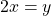The above equation is a merged form of the parametric equation:To merge a parametric equation into a single equation, rearrange the common parameter to one side. We shall put the parameter t on its own, on the right side.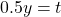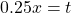Now equate both left sides. Hence,Multiply 4 to both sides to beautify it.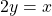## Why is it Important to You?

Parametric equations help us understand relationships between 2 parameters when they are related to other parameters.

In finance, we use parametric equations to understand the relationship between different financial products.

One use of parametric equations is to check the sensitivity of stocks against the overall market movement.

Knowing these sensitivities will allow us to size our bets when running a trading strategy that involves buying and shorting (To short a stock is to bet that it will drop).

One example is the pair trading strategy.

Sensitivity to the overall market

The overall market is a term that refers to the majority of stocks. This often refers to a group of stocks that represent the local stock market.

In the US, the most popular group of stocks is the S&P500. The S&P500 is a group of 500 major stocks in the US.

When the overall market moves, individual stocks will likely move in a similar manner.

However, each stock might move to a different extent.

Let’s assume that when the S&P500 moves by 1%, Tesla moves by 2% and General Motors (GM) moves by 0.5%.

Thus, the parametric equations are: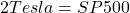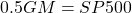The S&P500 is our common factor. Therefore: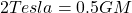Beautifying it…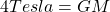We now know that a 4% move in Tesla is equivalent to a 1% move in GM.

Using this information in a Pair Trading Strategy

A pair trading strategy involves buying one stock and shorting another at the same time.

The idea of this strategy is to cancel out the exposure to the overall market while betting that one stock does better than the other.

If we were to buy Tesla and short GM, we could buy 1 share of Tesla and short 4 shares of GM.This way, if the S&P500 moves up 1%, we should gain 2% on our Tesla shares while the GM shares will will lose 2% in value (0.5% * 4 shares).

Netting them (2% – 2%) will result in no movement for our pair trade.

Our pair trade should not fluctuate when the overall markets move, but will work in our favor if Tesla’s business performs better than GM.

This is the bet we are making in this hypothetical scenario.